• +91 9971497814
• info@interviewmaterial.com

# Chapter 13- Boolean Algebra Interview Questions Answers

### Related Subjects

Question 1 :
Which gates are known as universal gates ? Why?

Universal gates are the ones which can be used for implementing any gate like AND, OR and NOT or any combination of these basic gates. NAND and NOR gates are universal gates.

Question 2 :
Draw the equivalent logic circuit for the following Boolean expression :

Answer 2 :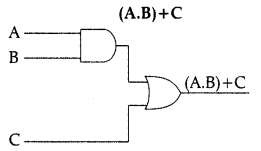Question 3 : Express the OR operator in terms of AND and NOT operator.

(A . B)’ =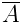+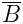(+)’ = A + B

Question 4 :
Specify which axioms/theorems are being used in the following Boolean reductions :
(a) (be)’ + be = 1
(b) xyz + zx = xz

(a) x + x’ = 1 & Complementary law
(b) y + x = x & Absorption law.

Question 5 : State and verify Associative law using Truth Table.

Answer 5 : Associative Law: This law states that:
(A + B) + C = A + (B + C)
(A.B).C = A. (B.C)
Proof: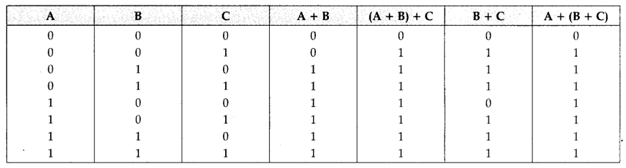From above truth table,
(A + B) + C = A + (B + C)
Similarly, we can prove,
A. (B.C) = (A. B).C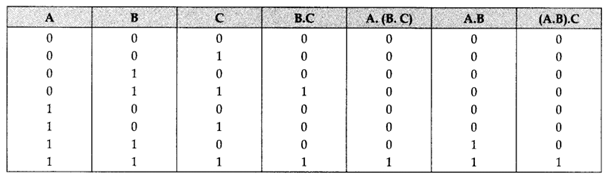Question 6 :
Correct the following boolean statements :
1. X+1 = X
2. (A’)’ = A’
3.  A+A’ = 0
4.  (A+B)’ = A.B

1. X+l=l or X+0=X
2. ((A’)’) = A
3. A + A’ = 1 or A. A’ = 0
4. (A 4- B)’ = A’.B1

Question 7 : Write the POS form of a Boolean Function F, which is represented in atruth table as follows :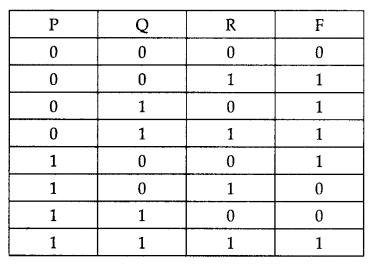(P+Q+R).(P’+Q+R).(P’+Q’+R)

Question 8 : State and Verify Absorption law algebraically.

Absorption law states that :
A + AB = A and A. (A + B) = A
Algebraic method :
Taking LHS
A + AB = (A.l) + (A.B) by Identity
= A. (1 + B) by Distribution
= A.l by Null Element
= A

Question 9 : State and define principle of duality. Why is it so important in Boolean Algebra ?

Principle of duality : Duality principle states that from every boolean relation another boolean relation can be derived by :
(i) Changing each OR sign (+) to an AND sign (-).
(ii) Changing each AND sign (-) to an OR sign (+)
ex : Dual of A + A’B = A. (A’ + B)
Importance in Boolean Algebra : The principle of duality is an important concept in Boolean algebra, particularly in proving various theorems. The principle of duality is used extensively in proving Boolean algebra theorem. Once we prove that an expression is valid, by the principle of duality, its dual is also valid. Hence, our effort in proving various theorems is reduced to half.

Question 10 : Name the law shown below & verify it using a . truth table.
X+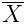.Y = X + Y.

This law is called “AbsorptionLaw” also referred as redundance law.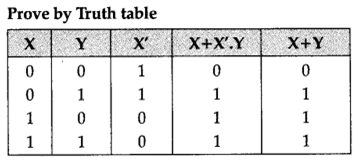Todays Deals### Chapter 13- Boolean Algebra Contributorskrishan

Name:
Email:

# Latest News# 9000 interview questions in different categories